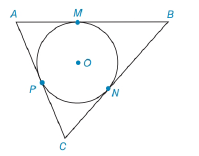Chapter 6.3, Problem 35E### Elementary Geometry for College St...

6th Edition
Daniel C. Alexander + 1 other
ISBN: 9781285195698

#### Solutions

Chapter
Section### Elementary Geometry for College St...

6th Edition
Daniel C. Alexander + 1 other
ISBN: 9781285195698
Textbook Problem
8 views

# Given: Tangents A B ¯ , B C ¯ ,     and     A C ¯     t o     ⊙ O at points M, N, and P, respectively. A B = 14 , B C = 16 , A C = 12 Find: AM, PC, and BNTo determine

To find:

To find AM, PC, and BN.

Explanation

Given that, Tangents AB¯,BC¯, and AC¯ to O at points M, N, and P, respectively AB=14,BC=16,AC=12

The tangents segments from an external point are congruent. Therefore,

AM=AP=xBM=BN=yCN=CP=z

The diagrammatic representation is given below,

We know that,

AB=AM+MB=x+yBC=BN+NC=y+zAC=AP+PC=x+z

Substitute the value of AB=14,BC=16,AC=12 in the above equation to get the following,

x+y=14...(1)y+z=16...(2)x+z=12...(3)

Solve equation (1) and (2) to get the following,

x+y+0z=140x+y+z=16()(

### Still sussing out bartleby?

Check out a sample textbook solution.

See a sample solution

#### The Solution to Your Study Problems

Bartleby provides explanations to thousands of textbook problems written by our experts, many with advanced degrees!

Get Started

#### What is the lowest score in the following distribution?

Essentials of Statistics for The Behavioral Sciences (MindTap Course List)

#### Find the domain of the function. 32. f(x)=2x35x2+x6

Single Variable Calculus: Early Transcendentals, Volume I

#### True or False: f(x) is symmetric about the origin if f(x) =f(x).

Study Guide for Stewart's Single Variable Calculus: Early Transcendentals, 8th

#### The graph at the right has equation:

Study Guide for Stewart's Multivariable Calculus, 8th